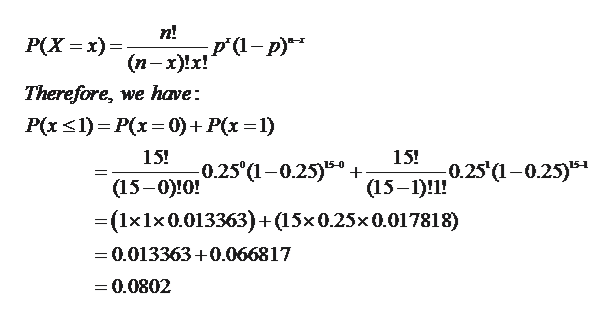# Find P(x <= 1 | n = 15, p = 0.25) by using the binomial formula.

Question
1 views
 Find P(x <= 1 | n = 15, p = 0.25) by using the binomial formula.
check_circle

Step 1

Solution: We are given that a random variable x follows the binomial distribution with n = 15 and p = 0.25.

Therefore, we are given:

Step 2

We have to find:

Step 3

Now using the binomial probability mass...help_outlineImage Transcriptionclosen! p'(1- p)* Р(X — х)— (п-х)!x! Therefore, we have: P(x<1) = P(x= 0)+ P(x =1) 15! 15! 0.25'(1-0.25) 0.25 (1-0.25)5"+ (15-0)!0! (15–1)!1! =(1x1x0.013363)+(15x0.25x0.017818) = 0.013363+0.066817 = 0.0802 fullscreen

### Want to see the full answer?

See Solution

#### Want to see this answer and more?

Solutions are written by subject experts who are available 24/7. Questions are typically answered within 1 hour.*

See Solution
*Response times may vary by subject and question.
Tagged in

### Measures